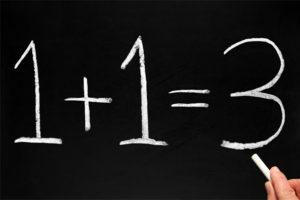Posted on

## Infallible Calculator?You may have seen the claim on the Internet that millions of people get the answer to the equation$6 \div 2\left(1 + 2\right)$  (or similar) wrong. You may have even read the explanations by some people about why the answer should be 9 or should be 1. Let me clear it up and explain why the answer is most definitely 1, but why the calculator (and others) give 9.

Firstly, the important thing to recognise is that this equation, like all others, is subject to the Order of Operations, which states that we must solve the contents of the brackets first. Thus our equation becomes$6 \div 2(3)$ .

Posted on